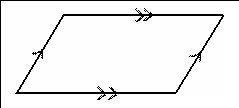• Shuffle
Toggle On
Toggle Off
• Alphabetize
Toggle On
Toggle Off
• Front First
Toggle On
Toggle Off
• Both Sides
Toggle On
Toggle Off
Toggle On
Toggle Off
Front

## Card Range To Study

throughPlay buttonPlay buttonProgress

1/16

Click to flip

Use LEFT and RIGHT arrow keys to navigate between flashcards;

Use UP and DOWN arrow keys to flip the card;

H to show hint;

 parallelogramWhat is this polygon? opposite sides are parallel 1 pair of opposite sides are parallel trapezoid diagonals are congruent rectangle diagonals bisect each other parallelogram diagonals are perpendicular rhombus diagonals are perpendicular and diagonals bisect each other square opposite sides are congruent and parallel parallelogram 4 pairs of congruent sides rhombus 4 right angles rectangle 4 right angles and 4 pairs of congruent sides square consecutive angles are supplementary parallelogram opposite angles are congruent parallelogram opposite sides are parallel and congruent parallelogram opposite sides are parallel and congruent AND 4 right angles rectangle take the number of sides and subtract 2. Multiply this number by 180 sum of the interior angles of a polygon take the number of sides and subtract 2. Multiply this number by 180. Then divide by the total number of sides. measure of each of the interior angles of a regular polygon (n sides)